# An inexact match can be exactly right!

Exact or inexact?

Firstly, let us be clear about the difference between exact and inexact matches.

Exact matches

Normally when you are doing a lookup using MATCH, XLOOKUP or VLOOKUP, you want to find an exact match in your look up table. For example, you want the cash discount percent for a specific customer. When you look up the customer account number in your lookup table, you want to find the result for the specific customer and not for another customer which happens to have a similar account number.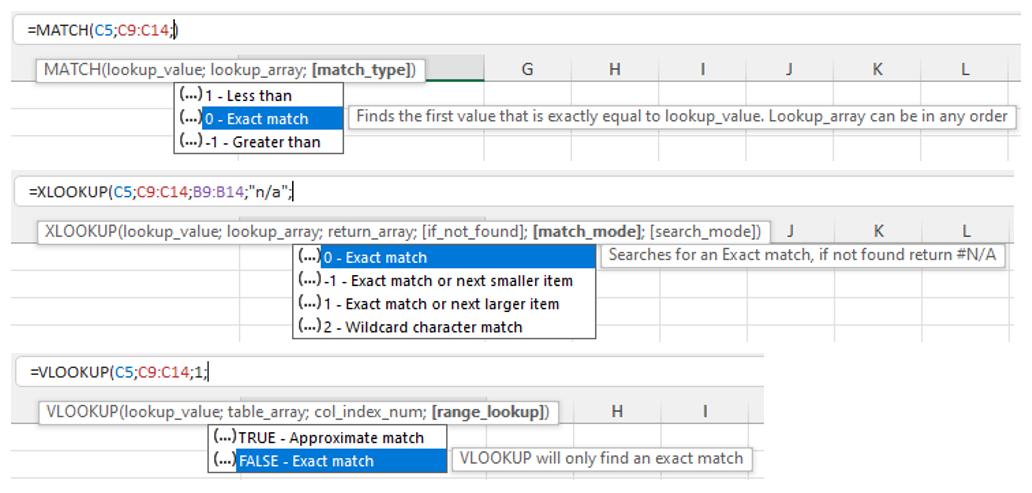In such cases you need to use the optional argument of 0 for MATCH and XLOOKUP or FALSE for VLOOKUP. The lookup table data can be in any order, Excel keeps looking until it finds the first match, or reports an error if it cannot find the searched item at all.

Inexact matches

In some cases, however, it may be necessary to use an inexact match if the item you are looking for may not be exactly found in the lookup table, for example a list of sales discounts based upon the quantity sold (see example 1 below).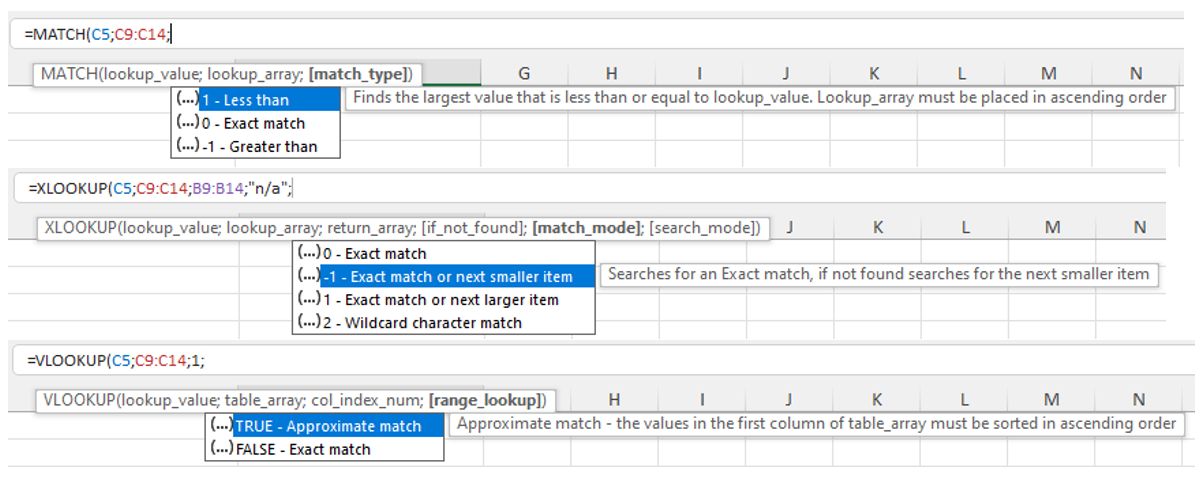In such cases you need to use the optional argument of 1 or -1 for MATCH and XLOOKUP or TRUE for VLOOKUP. The lookup table data must then be in ascending or descending order, per the tooltip shown (note that VLOOKUP can only cope with lookup data in ascending order). Excel then searches until it either finds an exact match or returns the result that is “not too far”. This is best illustrated with some examples.

In all four examples there is an input cell (grey background) and an output cell (green background). The output cell uses the powerful combination of the INDEX and MATCH functions to get the correct result from the lookup table using an inexact match. You could use XLOOKUP instead (or possibly even VLOOKUP but I do not recommend this function as it has a number of weaknesses and risks).

1. Sales discount
• Task: Input the quantity sold to get the discount percent.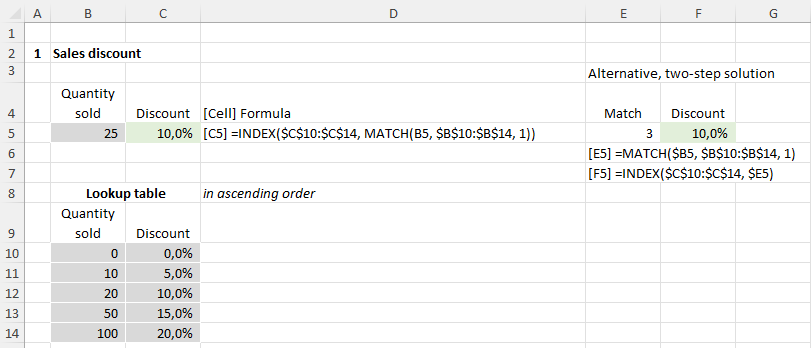• Solution: We need to find the largest “quantity sold” in the lookup table which is less than or equal to the input quantity to get the corresponding discount percent. We therefore use the inexact match argument 1.
• Lookup table: This requires that the look up table is sorted in ascending order.

• Task: Input the exam result in percent to get the exam grade.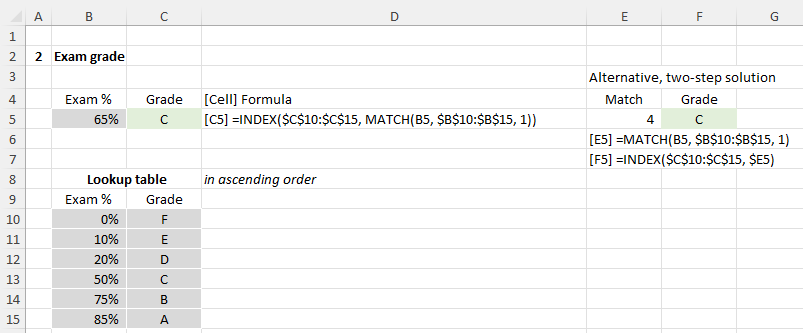• Solution: Similar to case 1. The inexact match argument is also 1
• Lookup table: Per case 1, sorted in ascending order.

1. Payment date
• Task: Input the invoice due date to get the next payment date.
This can be very useful for cash planning tools: use an accounts payable report to generate payment dates.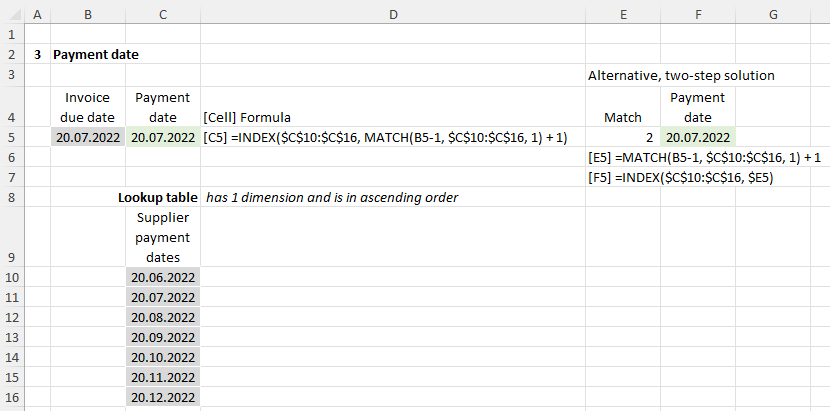• Solution: If the lookup table is in descending order, the solution is relatively straight forward, but in reality a list of dates will probably be in ascending order. This makes the solution a little trickier but with a bit of testing you can find the correct approach.
> Search date: We must deduct 1 from the input due date in order to also get the correct result when the input date exactly matches a payment date, otherwise we will get the following payment date.
> MATCH finds us the largest (i.e. latest) payment date which is less than or equal to the search date, i.e. it gives us the prior payment date. We must therefore add one to the MATCH result to get the next payment date.
• Lookup table: The lookup table is sorted in ascending order (because that is probably how it will be) but has only one dimension. The formula searches for the invoice due date (input) in the list of payment dates and returns the next payment date from the same column.

1. Fruit sorter
• Task: Lastly for a bit of fun we have a fruit sorter 😊
Input the fruit size in centimetres to find the smallest box that is big enough to hold it.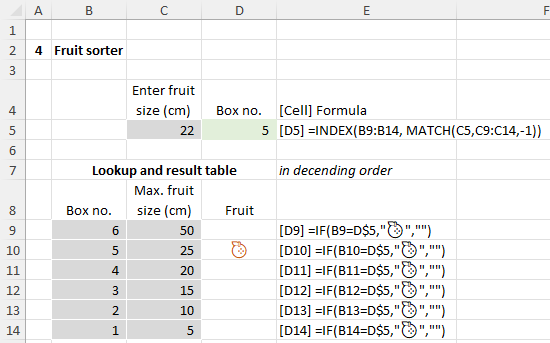• Solution: Because we are looking for the smallest box that is greater than or equal to the fruit size we need to use the inexact match argument -1.
• Lookup table: In this case, the lookup table must be sorted in descending order. There is an extra column here to clearly show the box in which the fruit ends up. This is determined using a simple IF formula with a fruit symbol… this is an emoji selected using the Windows key and . and then typing fruit to see a selection of fruit symbols. After my selection, I changed the text colour of the "Fruit" column to a dark orange.
• And action! Here's the solution in action...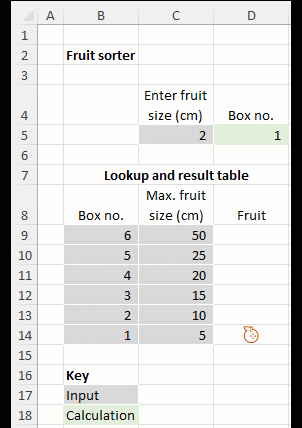You want more results?

These approaches are not restricted to a single output cell but can be used in a large table of calculations with results calculated for each row, as shown below for the fruit sorter with a chart of results for analysis.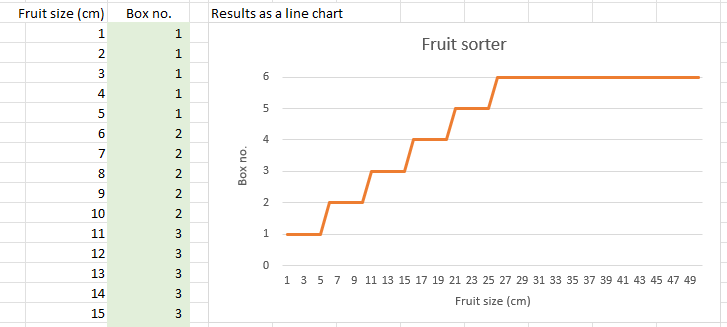I hope this blog helps you in your work!

More help

For more explanations please see my videos on INDEX and MATCH or XLOOKUP,  Here you will also learn the problems and risks associated with VLOOKUP.